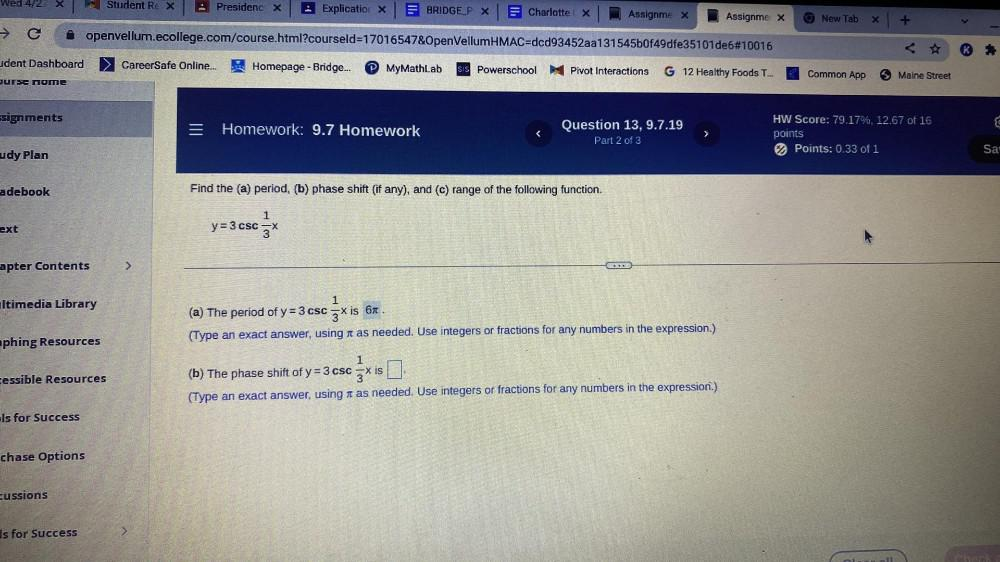Question:

# Find the (a) period, (b) phase shift (if any), and (c) range of the following function. y = 3csc⅓x (a) The period of y = 3csc⅓x is (Type an exact answer, using a as needed. Use integeFind the (a) period, (b) phase shift (if any), and (c) range of the following function. y = 3csc⅓x (a) The period of y = 3csc⅓x is (Type an exact answer, using a as needed. Use integers or fractions for any numbers in the expression.) (b) The phase shift of y = 3csc⅓x is (Type an exact answer, using π as needed. Use integers or fractions for any numbers in the expression.)